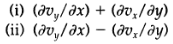### Create an Account

Home / Questions / A fluid in a state of rigid rotation a Verify that the velocity distribution c in Problem ...

# A fluid in a state of rigid rotation a Verify that the velocity distribution c in Problem 1B 1 describes a fluid in a state of pure rotation that is the fluid is rotating like a rigid body What

A fluid in a state of rigid rotation
(a) Verify that the velocity distribution (c) in Problem 1B.1 describes a fluid in a state of pure rotation; that is, the fluid is rotating like a rigid body. What is the angular velocity of rotation?
(b) For that flow pattern evaluate the symmetric and anti symmetric combinations of velocity derivatives:
(c) Discuss the results of (b) in connection with the development in S1.2.Jul 25 2020 View more View LessSubscribe To Get Solution